Test: Factors & Multiples

# Test: Factors & Multiples

Test Description

## 20 Questions MCQ Test Mathematics for Class 5: NCERT | Test: Factors & Multiples

Test: Factors & Multiples for Class 5 2023 is part of Mathematics for Class 5: NCERT preparation. The Test: Factors & Multiples questions and answers have been prepared according to the Class 5 exam syllabus.The Test: Factors & Multiples MCQs are made for Class 5 2023 Exam. Find important definitions, questions, notes, meanings, examples, exercises, MCQs and online tests for Test: Factors & Multiples below.
Solutions of Test: Factors & Multiples questions in English are available as part of our Mathematics for Class 5: NCERT for Class 5 & Test: Factors & Multiples solutions in Hindi for Mathematics for Class 5: NCERT course. Download more important topics, notes, lectures and mock test series for Class 5 Exam by signing up for free. Attempt Test: Factors & Multiples | 20 questions in 20 minutes | Mock test for Class 5 preparation | Free important questions MCQ to study Mathematics for Class 5: NCERT for Class 5 Exam | Download free PDF with solutions
 1 Crore+ students have signed up on EduRev. Have you?
Test: Factors & Multiples - Question 1

### Which of the following statements is correct?

Test: Factors & Multiples - Question 2

### Find the number of multiples of 5.

Test: Factors & Multiples - Question 3

### How many prime numbers are there "between 1 to 100"?

Detailed Solution for Test: Factors & Multiples - Question 3

Prime numbers are 2, 3, 5, 7, 11, 13, 17, 19, 31, 37, 41, 43, 47, 53, 59, 61, 67, 71, 73, 79, 83, 89, 97 = 25

Test: Factors & Multiples - Question 4

Which of the following is a perfect square?

Detailed Solution for Test: Factors & Multiples - Question 4

25 is the perfect square of 5. rest all options are not perfect squares of any natural number.

Test: Factors & Multiples - Question 5

What is the HCF of two prime numbers?

Test: Factors & Multiples - Question 6

What is the LCM of 36, 75 and 80?

Detailed Solution for Test: Factors & Multiples - Question 6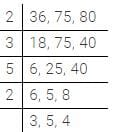LCM = 2×3×5×2×3×5×4 = 3600

Test: Factors & Multiples - Question 7

Product of two numbers = ___________

Test: Factors & Multiples - Question 8

The sum of the factors of 25 is

Detailed Solution for Test: Factors & Multiples - Question 8

Factors of = 25 = 52 are 1, 5, 25 which can also be represented as: 5= (2+1) = 3 (i.e.3 factors)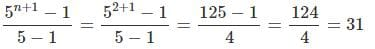Test: Factors & Multiples - Question 9

A number is divisible by 24 if ___________

Detailed Solution for Test: Factors & Multiples - Question 9

It is divisible by 8 & 3 because 8 and 3are co-prime

Test: Factors & Multiples - Question 10

Prime factorization of 512 × 343

Detailed Solution for Test: Factors & Multiples - Question 10

512×343 =

2×2×2×2×2×2×2×2×2×7×7×7=29 x 73

Test: Factors & Multiples - Question 11

Determine the number nearest to 100000 but greater than 100000 which is exactly divisible by each of 8, 15 and 21?

Detailed Solution for Test: Factors & Multiples - Question 11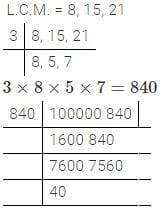Reminder= 40 84040=800 100000+800=100800

Test: Factors & Multiples - Question 12

182/195 when reduced to the lowest term is

Detailed Solution for Test: Factors & Multiples - Question 12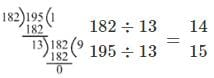Test: Factors & Multiples - Question 13

Which of the following pair is co-prime?

Detailed Solution for Test: Factors & Multiples - Question 13

HCF of 81 and 135 = 27 HCF of 87 and 116 = 29 HCF of 43 and 73 = 1 HCF of 26 and 65 = 13  Hence, 43 and 73 are co-prime

Test: Factors & Multiples - Question 14

Find the number of factors of 1024

Detailed Solution for Test: Factors & Multiples - Question 14

1024=210=2n
⇒ (n+1) = (10+1) = 11.
Where (n+1) =  no. of factors. Here factors will be 1, 2, 4, 8, 16?????.1024.

Test: Factors & Multiples - Question 15

If the 8-digit number 136y5785 is divisible by 15, then find the least possible value of

Detailed Solution for Test: Factors & Multiples - Question 15

136Y5785

For 15, No. should be divisible by 5, 3
No. is already divisible by 5 1 + 3 + 6 + 5 + 7 + 8 + 5 + y = 35 + y

∴y=1

Test: Factors & Multiples - Question 16

Find the smallest number which when divided by 25, 40 and 60 leaves remainder 7 in each case?

Detailed Solution for Test: Factors & Multiples - Question 16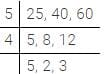5×4×5×2×3=600
Required numbers is 600+7=607

Test: Factors & Multiples - Question 17

x, y, z, w are four odd natural numbers. Let u=x2+y2+z2+w2. Consider following statements

I. U is always divisible by 4
II. U is never divisible by 8

Q. Which of the above statements) is /are true?

Test: Factors & Multiples - Question 18

2211/5025 , which reduced to its simplest form. By dividing the numerator and denominator

Detailed Solution for Test: Factors & Multiples - Question 18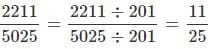Test: Factors & Multiples - Question 19

A boy saves Rs. 4.65 daily. Find the least number of days in which he will be to save an exact number (whole number) of rupees.

Detailed Solution for Test: Factors & Multiples - Question 19

Rs. 4.65 = 465 paise

The exact number of rupees will be a multiple of 100

So, we have to fine the L.C.M. of 465 and 100

100 = 2*2*5*5

465 = 3*5*31

L.C.M. of 465 and 100 = 2*2*3*5*5*31

= 9300

So, the boy saves 9300 paise

Hence, least number of days required to save 9300 paise = 9300/465

= 20 days

Test: Factors & Multiples - Question 20

H.C.F of two numbers is 16, then their LCM is

Detailed Solution for Test: Factors & Multiples - Question 20

H.C.F is always a factor of L.C.M. In this case 16 Divides 192 only.

## Mathematics for Class 5: NCERT

29 videos|73 docs|45 tests
Information about Test: Factors & Multiples Page
In this test you can find the Exam questions for Test: Factors & Multiples solved & explained in the simplest way possible. Besides giving Questions and answers for Test: Factors & Multiples, EduRev gives you an ample number of Online tests for practice

## Mathematics for Class 5: NCERT

29 videos|73 docs|45 tests(Scan QR code)